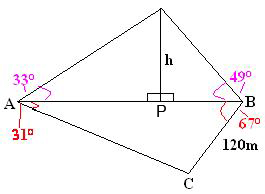Quandaries and Queries hi, my name is Vinita my email address is as the following i am a student whose currently in grade 11 in university and college math(mixed) i am having a complex time in solving this question. Observers at point A and B, who Stand on level ground on opposite sides of a tower, measure the angle of elevation to the top of the tower to be 33 degrees and 49 degrees respectively. Another point C is 120 m from point B, Triangle ABC =67 degrees and BAC = 31 degrees. Find the height of the tower to the nearest metre. Hi Vinita, I copied the diagram you sent and added a P, a name for the point on the ground at the bottom of the tower.Since you know the angle ACB is 180 - (67 + 31) = 82 degrees you can use the Law of Sines with triangle ACB to find the distance between A and B. Call this distance d meters. Let x be the distance in metres between P and B and then the distance from A to P is d - x meters. From this you know tan(330) = h/(d-x) and tan(490) = h/x Solve for h. Penny